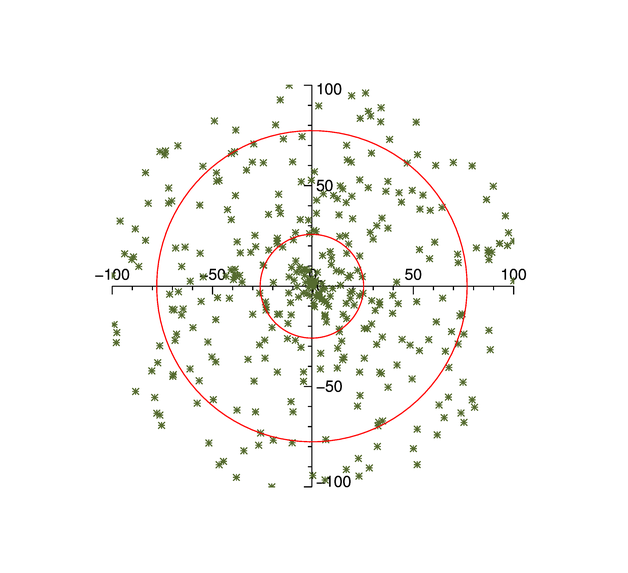# Creating a Polar Plot

QUESTION: Donald Stuart Finan of Indiana University wrote in the IDL newsgroup recently:

Am I missing something or is there no easy way to do polar plots (with 0-360 deg axes) in IDL? All I want to do is to make a phase plot with concentric rings for the radius. This is my first experience with polar plots, so it's slow going. Any tips?ANSWER: I wrote a little example program, named PolarPlotCenter, for Donald. For the concentric rings I used a program named Circle, which I downloaded from this web page.

The PolarPlotCenter program is a wrapper for the cgPlot command and actually uses the cgPlot command with the Polar keyword set to create the polar coordinate space. The normal plot axes are supressed and the polar axes are drawn through the center of the polar plot with the cgAxis command. The code for the program looks like this:

```    PRO PolarPlotCenter, radius, angle

; Fake data if needed.
IF N_Params() EQ 0 THEN BEGIN
angle = ((Randomu(seed, 360)*360) - 180) * !DtoR
radius = Randomu(seed, 360) * 100
ENDIF

; Establish plot coordinates
cgPlot, radius, angle, /Polar, XStyle=5, YStyle=5, \$
/NoData, Aspect=1.0

; Draw axis through center.
cgAxis, /XAxis, 0, 0
cgAxis, /YAxis, 0, 0

; Plot data.
cgPlot, radius, angle, PSym=2, Color='olive', /Overplot, /Polar

; Draw 25 and 75 percent circles.
percent25 = Circle(0, 0, 0.25*dataMax)
percent75 = Circle(0, 0, 0.75*dataMax)
cgPlotS, percent25, Color='red'
cgPlotS, percent75, Color='red'

END
```

To run the program, first download the program listed above and type this:

```   IDL> PolarPlotCenter
```

You see the results of the program in the illustration below.Here is an example of the PolarPlotCenter program above called with the default data set. Concentric circles represent 25% and 75% of the maximun data value. Axes are drawn through the center of the plot.A polar plot with concentric circles and axes through the center of the plot.